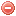# Description

The Portuguese index was developed by the Portuguese meteorological and geophysical national institute based on the Nesterov index (Instituto Nacional de Meteorologia e Geofisica 1988, Gonçalves & Lourenço 1990, Camia & Bovio 2000), and requires air temperature, dew point temperature and wind speed at noon, as well as daily rainfall as input data.

The Portuguese index is composed of three numerical indicators (Camia & Bovio 2000): (1) a daily ignition index; (2) a cumulative index consisting of the sum of the ignition index previous days' values (from the beginning of the fire season), and corrected by a factor depending on the previous day's rainfall; and (3) the final fire danger index which consists of the combination of (1) and (2), and is corrected for wind speed.

# Formula

The ignition index $$IG$$ on day $$t$$ is calculated as follows:

$IG_t=T_{12_t}\cdot(T_{12_t}-T_{dew_{12_t}})$

where $$T_{12}$$ is air temperature [°C] and $$T_{dew_{12}}$$ dew point temperature [Â°C] at noon.

The cumulative index $$B$$ on day $$t-1$$ is calculated as follows:

$B_{t-1}=p_{t-1}\displaystyle \sum_{i=1}^{t-1} IG_i$

where $$p$$is the correction factor for the previous day's rainfall $$P$$, which is determined according to the following table:

p Rainfall [mm]
1 0 < R ≤ 1
0.8 1 < R ≤ 2
0.6 2 < R ≤ 3
0.4 3 < R ≤ 4
0.2 4 < R ≤ 10
0.1 R > 10

The fire danger index $$Ifa$$ on day $$t$$ is finally calculated as follows:

$Ifa_t=IG_t+B_{t-1}$

Note: the original publication is still not gathered. According to the Megafires project following conversion should be performed before calculating $$Ifa$$:

IG New value
IG < 151 1
151 ≤ IG < 301 2
301 ≤ IG < 451 3
451 ≤ IG < 601 4
601 ≤ IG < 751 5
IG >= 751 6

B New value
B ≤ 301 1
301 ≤ B < 1001 2
1001 ≤ B < 2001 3
2001 ≤ B < 4001 4
4001 ≤ B < 6001 5
6001 ≤ B < 8001 6
8001 ≤ B < 10001 7
10001 ≤ B < 12001 8
12001 ≤ B < 15001 9
15001 ≤ B < 18001 10
18001 ≤ B < 21001 11
21001 ≤ B < 24001 12
24001 ≤ B < 27001 13
27001 ≤ B < 31001 14
31001 ≤ B < 35001 15
35001 ≤ B < 39001 16
39001 ≤ B < 43001 17
43001 ≤ B < 47001 18
47001 ≤ B < 51001 19
B >= 51001 20

Ifa has to be corrected for wind speed V as follows:

Wind speed [km/h] Correction to Ifa
V ≤ 10 none
10 < V ≤ 15 +1
15 < V ≤ 20 +2
20 < V ≤ 30 +3
30 < V ≤ 40 +4
V > 40 +5

# Index interpretation

according to megafires project. Still not clear if also proposed in the original publication

Corrected Ifa Danger class
0-1 I None
2-4 II Low
5-8 III Moderate
9-12 IV High
13-17 V Severe
>18 VI Extreme

Original publication:
Instituto Nacional de Meteorologia e Geofisica 1988
Gonçalves & Lourenço 1990
Other publication:
Viegas et al. 1999
Camia & Bovio 2000
Fujioka et al. 2008

### Bibliography SearchNo results!

No results were found. Are you sure you searched for a tag?

### Symbols

 Variable Description Unit $$T$$ air temperature °C $$T_{dew}$$ dew point temperature °C $$H$$ air humidity % $$P$$ rainfall mm $$U$$ windspeed m/s $$w$$ days since last rain (or rain above threshold) d $$rr$$ days with consecutive rain d $$\Delta t$$ time increment d $$\Delta{e}$$ vapor pressure deficit kPa $$e_s$$ saturation vapor pressure kPa $$e_a$$ actual vapor pressure kPa $$p_{atm}$$ atmospheric pressure kPa $$PET$$ potential evapotranspiration mm/d $$r$$ soil water reserve mm $$r_s$$ surface water reserve mm $$EMC$$ equilibrium moisture content % $$DF$$ drought factor - $$N$$ daylight hours hr $$D$$ weighted 24-hr average moisture condition hr $$\omega$$ sunset hour angle rad $$\delta$$ solar declination rad $$\varphi$$ latitude rad $$Cc$$ cloud cover Okta $$J$$ day of the year (1..365/366) - $$I$$ heat index - $$R_n$$ net radiation MJ⋅m-2⋅d-1 $$R_a$$ daily extraterrestrial radiation MJ⋅m-2⋅d-1 $$R_s$$ solar radiation MJ⋅m-2⋅d-1 $$R_{so}$$ clear-sky solar radiation MJ⋅m-2⋅d-1 $$R_{ns}$$ net shortwave radiation MJ⋅m-2⋅d-1 $$R_{nl}$$ net longwave radiation MJ⋅m-2⋅d-1 $$\lambda$$ latent heat of vaporization MJ/kg $$z$$ elevation m a.s.l. $$d_r$$ inverse relative distance Earth-Sun - $$\alpha$$ albedo or canopy reflection coefficient - $$\Delta$$ slope of the saturation vapor pressure curve kPa/°C $$Cc$$ cloud cover eights $$ROS$$ rate of spread m/h $$RSF$$ rate of spread factor - $$WF$$ wind factor - $$WRF$$ water reserve factor - $$FH$$ false relative humidity - $$FAF$$ fuel availability factor - $$PC$$ phenological coefficient -

 Suffix Description $$-$$ mean / daily value $$_{max}$$ maximum value $$_{min}$$ minimum value $$_{12}$$ value at 12:00 $$_{13}$$ value at 13:00 $$_{15}$$ value at 15:00 $$_{m}$$ montly value $$_{y}$$ yearly value $$_{f/a}$$ value at fuel-atmosphere interface $$_{dur}$$ duration $$_{soil}$$ value at soil level

 Constant Description $$e$$ Euler's number $$\gamma$$ psychrometric constant $$G_{SC}$$ solar constant $$\sigma$$ Stefan-Bolzmann constant